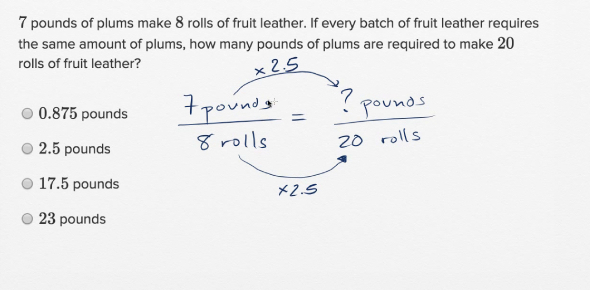# Could You Pass This Toughest SAT Math Practice Questions Test? Trivia Quiz

43 QuestionsSettingsSAT Math practice questions: This is an extensive, 50 SAT Math practice question quiz that is comprehensive over the subjects of algebraic manipulation, absolute value, inequalities, counting principles, logical reasoning, probability, and simple word problems. It is modelled on actual SAT Math section questions. So, let's try out the quiz. All the best!

Related Topics